# 2. Examples

These are example scripts using the py-pde package, which illustrates some of the most important features of the package.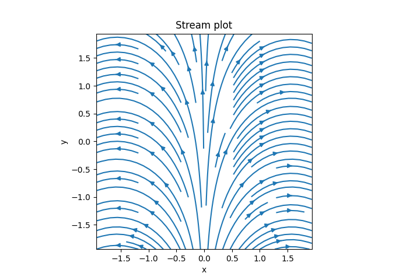Plotting a vector field

Plotting a vector field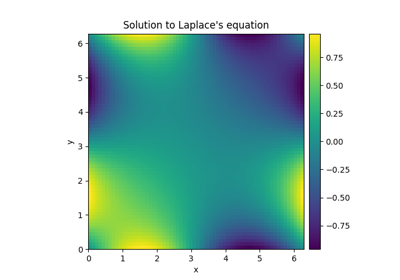Solving Laplace’s equation in 2d

Solving Laplace's equation in 2d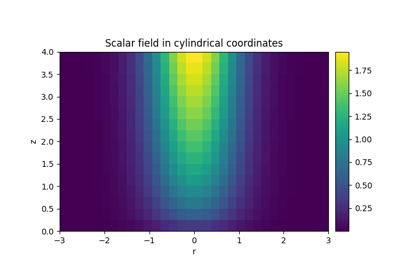Plotting a scalar field in cylindrical coordinates

Plotting a scalar field in cylindrical coordinates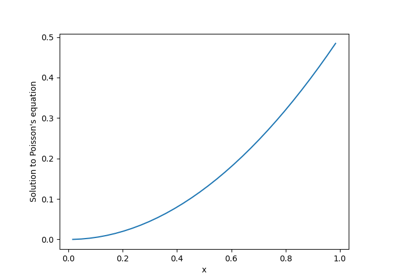Solving Poisson’s equation in 1d

Solving Poisson's equation in 1d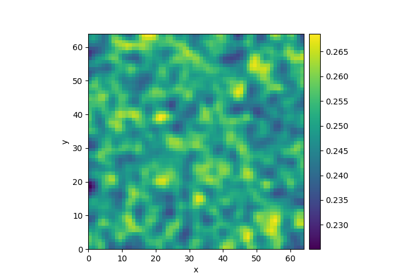Simple diffusion equation

Simple diffusion equation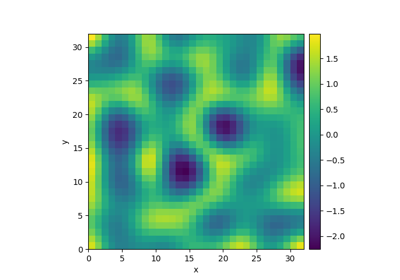Kuramoto-Sivashinsky - Using PDE class

Kuramoto-Sivashinsky - Using PDE class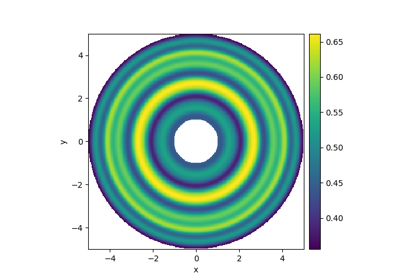Spherically symmetric PDE

Spherically symmetric PDE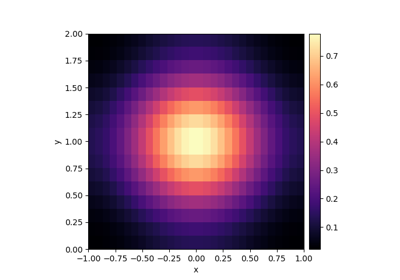Diffusion on a Cartesian grid

Diffusion on a Cartesian grid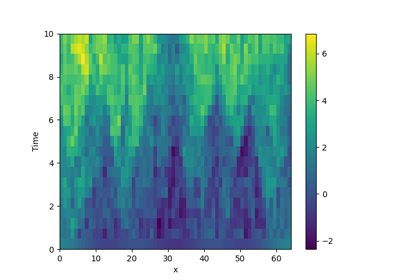Stochastic simulation

Stochastic simulation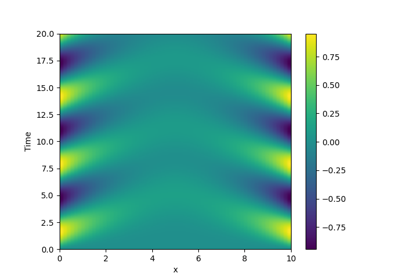Time-dependent boundary conditions

Time-dependent boundary conditions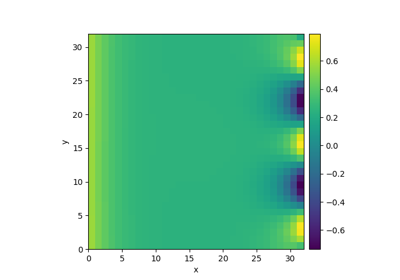Setting boundary conditions

Setting boundary conditions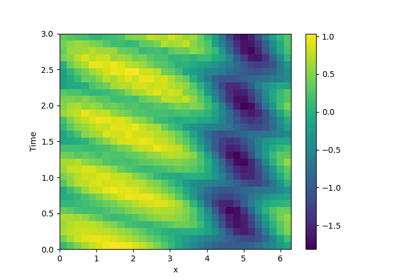1D problem - Using PDE class

1D problem - Using PDE class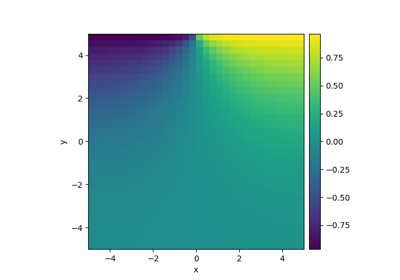Heterogeneous boundary conditions

Heterogeneous boundary conditions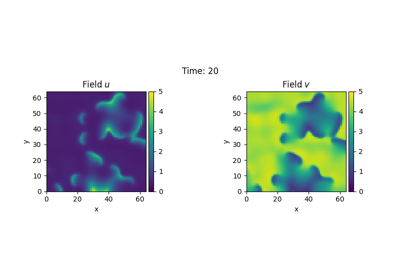Brusselator - Using the PDE class

Brusselator - Using the PDE class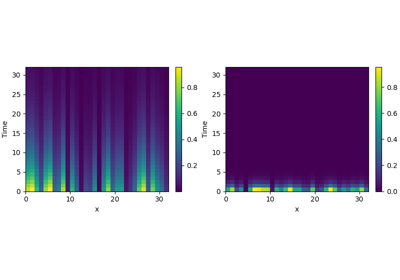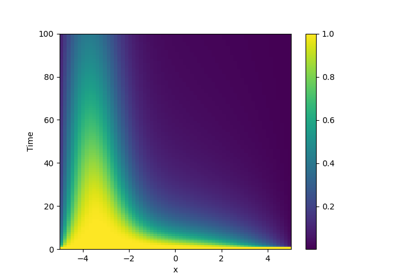Diffusion equation with spatial dependence

Diffusion equation with spatial dependence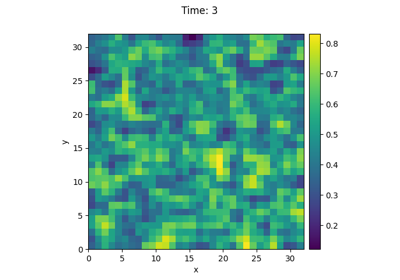Using simulation trackers

Using simulation trackers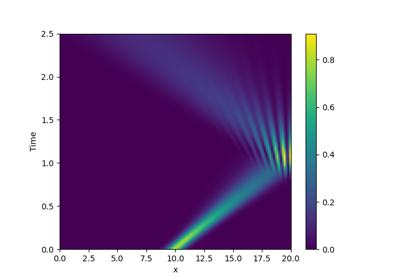Schrödinger’s Equation

Schrödinger's Equation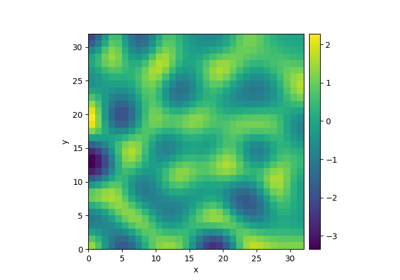Kuramoto-Sivashinsky - Using custom class

Kuramoto-Sivashinsky - Using custom class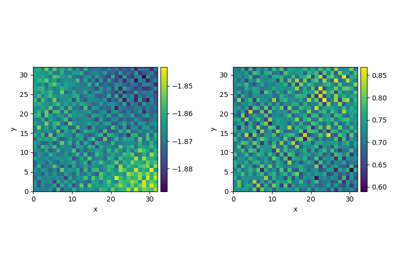Custom Class for coupled PDEs

Custom Class for coupled PDEs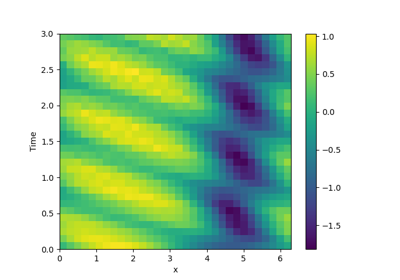1D problem - Using custom class

1D problem - Using custom class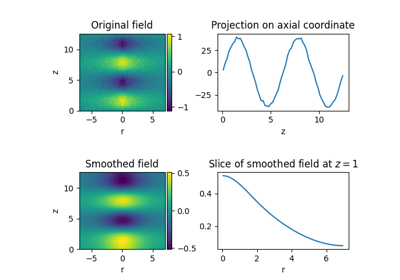Visualizing a scalar field

Visualizing a scalar field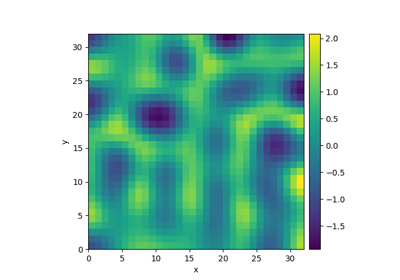Kuramoto-Sivashinsky - Compiled methods

Kuramoto-Sivashinsky - Compiled methods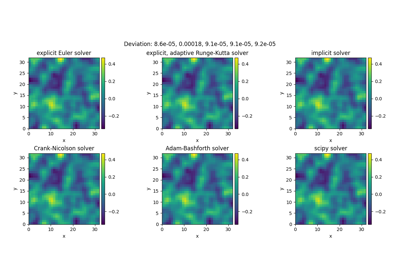Solver comparison

Solver comparison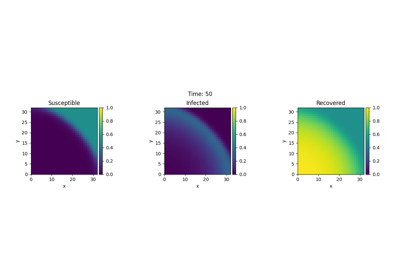Custom PDE class: SIR model

Custom PDE class: SIR model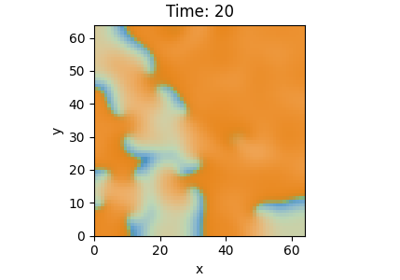Brusselator - Using custom class

Brusselator - Using custom class